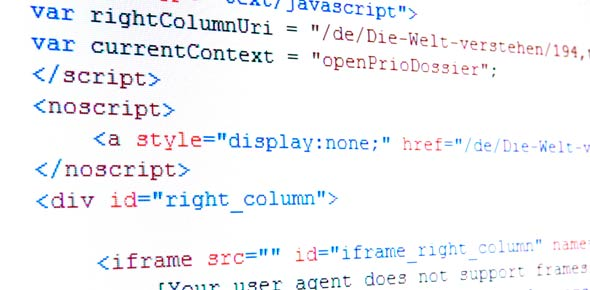# Ruby Programming Training Quiz #1

15 Questions | Attempts: 453
ShareSettings• 1.
Ruby array can contain values of which type?
• A.

Integers

• B.

Floats

• C.

Characters

• D.

Any type

• E.

Strings

• 2.
What is the size of Ruby array
• A.

10

• B.

A Ruby array has no fixed size

• C.

Count

• D.

None of the above

• 3.
What is the first index of an array
• A.

1

• B.

0

• C.

-1

• D.

None of the above

• 4.
Let's create an array:a=[1,2,3,4,5]Which statement creates the following output:[1, 2, 3, 4, 5]
• A.

Puts a[0..5]

• B.

A=[0,1,2,3,5] puts a[0..5]

• C.

Print a[1..5]

• D.

For count in a print a[count] end

• E.

None of the above

• 5.
Let's create an array:a=[12345, 23456, 34567, 98765]Which statement will print an index of the third element of this array.
• A.

A

• B.

Puts a

• C.

For count in a print count if count ==2 end

• D.

For count in a print a end

• 6.
What is the difference between a hash and an array in Ruby?
• A.

Hash indices can be of any type

• B.

There is no difference

• C.

The size of a hash is not fixed

• D.

The hash size is incremented dynamically

• E.

None of the above

• 7.
What is the name of key/value pair in Ruby hash?
• A.

Index

• B.

Value

• C.

Entry

• D.

Parameter

• E.

None of the above

• 8.
Which statement correctly defines hash h?
• A.

H=[fish => Catfish, dog => Terrier, cat => Siamese]

• B.

H={fish => Catfish, dog => Terrier, cat => Siamese}

• C.

H=[fish - Catfish, dog - Terrier, cat - Siamese]

• D.

H=[fish => Catfish dog => Terrier cat => Siamese]

• E.

None of the above

• 9.
Let's define a hash h. h={"fish" => "Catfish", "dog" => "Terrier", "cat" => "Siamese"} What is the right way to refer to the second element of this hash?
• A.

H

• B.

H

• C.

H[dog]

• D.

H['dog']

• E.

H["dog"]

• 10.
Let's define a hash h. h={"fish" => "Catfish", "dog" => "Terrier", "cat" => "Siamese"} Which for loop produces an error?
• A.

For animal in h puts animal end puts h['cat']

• B.

For animal, type in h puts "I love " + type + " " +animal +"s" end puts h['cat']

• C.

For count in h puts "I love " + type + " " +animal +"s" end puts h['cat']

• D.

For h in h puts h end

• E.

For h in h puts h end puts h['cat']

• 11.
What will a standard output of this code snippet be?name = "Alva"print name if name = 'Ary ' ; print "Alva" ;
• A.

AryAlva

• B.

Ary Alva

• C.

Nothing

• D.

Ary Alva

• E.

None of the above

• 12.
What will a standard output of this code snippet be?def print_parameters(par1, par2, par3)        puts par1        puts '#{par2}'        puts "#{par3}"end
• A.

Par1 par3

• B.

Par1 #{par2} par3

• C.

Par1 par2 par3

• D.

No output

• E.

None of the above

• 13.
Please look at this snippet of code:animal_sounds = {"dogs" => "bark", "elefant" => "trumpet", "lion" =>"roar", "cat" => "meow"}def zoo(param)        for each_animal, each_sound in param              puts each_sound        end        i_go ="Enough already!"endmy_pet_goes = zoo(animal_sounds)What is the value of my_pet_goes variable in the snippet of code below?
• A.

• B.

Roar

• C.

Bark

• D.

Trumpet

• E.

None of the above

• 14.
What makes a snippet of code into a block?
• A.

Putting square braces before the first statement and after the last statement of the snippet of code turns it into a block.

• B.

Making a method out of snippet of code turns it into a block.

• C.

Putting the word "do" at the beginning of the first statement and the word "end" after the last statement of the snippet of code turns it into a block.

• D.

Putting curly braces before the first statement and after the last statement of the snippet of code turns it into a block.

• E.

None of the above

• 15.
What is correct way to pass parameters to a block?
• A.

Block parameters are located at the beginning of the block, enclosed in a pipe symbols (||) and are separated by a colon.

• B.

Block parameters are located at the beginning of the block, enclosed in a pipe symbols (||) and are separated by space.

• C.

Block parameters are located at the beginning of the block, enclosed in square brackets and are separated by semicolon.

• D.

Block parameters are located at the beginning of the block and separated by semicolon.

• E.

None of the above

## Related TopicsBack to top
×

Wait!
Here's an interesting quiz for you.